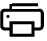# A Self Learning Tool - Math Times Tables Quiz Generator#Times Table Quiz Generator + Printable

An online printable mathematics times table quiz.### What are the benefits of a Times Table quiz?

(Self-Learning Tool)

mymathtables provides a wide range of workshops and math quizzes for Kids, school students and childrens to help improving math skills. Here are some ideas to make practicing with your child at home. Participate in online math quiz and see how they are performing with this math section and also there is an option to validate the answer and generate the new quiz.

Find free Math quizzes that will test your math skills and ability in areas such as basic addition, subtraction, multiplication, division, decimals and more.Enjoy all our printable quizzes as well as some interactive ones and have fun learning about math here at Kids Math Online.

Online test and printable math QUIZ will help students to improve their mathematical skills through this exercise, quizzes and tests. Students can select the level of questioning to practice and they can validate the answers also, score will be displayed to indicate a students level of understanding on this quiz.

###Related Quiz Generator ►

Interactive Times Table Quiz Generator

Times Table Generator PDF

###Math Charts ►

Geometric Shapes Chart

Multiplication Chart

##Math number calculations ►